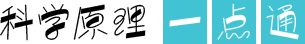360搜索与该网页作者无关，不对其内容负责。202111/03 10:57:00

# 特殊函数--周期函数

周期函数是一类重要的特殊函数，其性质受到广泛关注。主要研究集中在其最小正周期的存在性、周期函数和函数的周期性等问题。

周期函数的定义:一般地，对于函数f(x)，如果存在一个非零常数T，使得当x取定义域内每一个值时，都有f(x+T)=f(x)，那么函数f(x)就叫做周期函数，非零常数T叫做这个函数的周期。根据该定义可得到以下两个性质:

(1)如果f(x)定义在R上，且满足f(x+a)=f(x+b)，那么f(x)是|a-b| (a-b≠0) 为周期的周期函数

证明因为f(x+a)=f(x+b)，所以x用x-b代换得:f(x+a-b)=f(x)，故命题成立。

(2)如果f(x)定义在R上，且满足f(x+a)=-f(x+b)，那么f(x)是2|a-b| (a-b≠0) 为周期的周期函数

证明因为f(x+a)=-f(x+b)，所以x用x-b代换得:f(x+a-b) =-f(x)，x再用x+a-b代换得:f(x+2a-2b)=-f(x+a-b)，因此f(x+2a-2b)=f(x)故命题成立。

学习周期函数时，经常会遇到以下几个问题:

问题1:函数的周期都是指函数的最小正周期吗?

我们知道，对于函数f(x)，如果存在一个非零常数T，使得当x取定义域内的每一个值时，都有f(x+T)=f(x)，那么函数f(x)就叫周期函数，非零常数T则称为这个函数的周期。一般地，如果不加特别说明，我们都可以认为周期T是指函数的最小正周期。但若f(x+T)=f(x)，此时的T并不一定是函数f(x)的周期。

例如sin(x+π)=sin x对于x=0，π，2π，…都成立，但π并不是f(x)=sin x的周期，因为当x=时，sin(x+π)≠sin x,f(x)=sin x的周期实际上为2π。

同时，还有些周期函数不存在最小正周期。如狄里克雷函数D(x)=是没有最小正周期的函数。

问题2:求函数周期的常用方法有哪些?

求周期常用的方法有:定义法，公式法，图象法。

定义法较为简单，我们直接利用定义使f(x+T)=f(x)，即可得出函数的周期。

公式法主要是利用公式T=求出函数的周期。

图像法可以通过下边一个例题来帮助我们了解周期函数例:求f(x)=||的周期。

解:绘制f(x)=||的函数图图像，由图可知函数的周期为。

本文由石家庄市藁城区第九中学一级教师刘丽芳进行科学性把关。